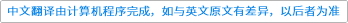International Journal of Foundations of Computer Science ( IF 0.523 ) Pub Date : 2021-01-04 , DOI: 10.1142/s0129054121500064
Ting Yao; Shixin Zhu; Binbin Pang

Let $R=𝔽q+u𝔽q$, where $q$ is a power of a prime number $p$ and $u2=0$. A triple cyclic code of length $(r,s,t)$ over $R$ is a set that can be partitioned into three parts that any cyclic shift of the coordinates of the three parts leaves the code invariant. These codes can be viewed as $R[x]$-submodules of $R[x]/〈xr−1〉×R[x]/〈xs−1〉×R[x]/〈xt−1〉$. In this paper, we study the generator polynomials and the minimum generating sets of this kind of codes. Some optimal or almost optimal linear codes are obtained from this family of codes. We present the relationship between the generators of triple cyclic codes and their duals. As a special class of triple cyclic codes, separable codes over $R$ are discussed briefly in the end.𝔽q+u𝔽q上的三重循环码

$[R=𝔽q+ü𝔽q$，在哪里 $q$ 是素数的幂 $p$$ü2=0$。长度为三重循环码$（[R，s，Ť）$ 过度 $[R$是一个可以分为三部分的集合，这三部分的坐标的任何循环移位都会使代码不变。这些代码可以视为$[R[X]$-的子模块 $[R[X]/〈X[R-1个〉×[R[X]/〈Xs-1个〉×[R[X]/〈XŤ-1个〉$。在本文中，我们研究了这类代码的生成多项式和最小生成集。从该系列代码中获得一些最佳或几乎最佳的线性代码。我们介绍了三重循环码的生成器与其对偶之间的关系。作为三重循环码的特殊类别，$[R$ 最后简要讨论。

down
wechat
bug